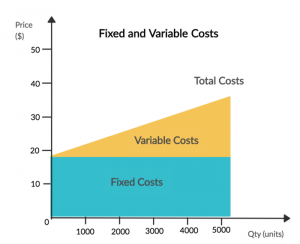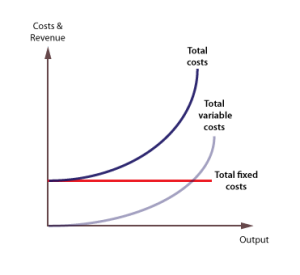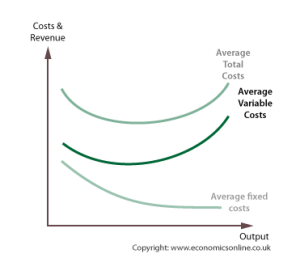# How to calculate the variable cost? Follow these steps

Understanding business finance is imperative for the success of every enterprise. Before you delve into terms like cash flow and inventory management, you must first begin with the basics. It includes fixed and variable costs.

People tend to make the mistake of only considering the fixed costs when gauging the feasibility of a project. However, certain costs rise as the business undergoes expansion. These are the variable cost and are much more critical when deciding the quantity of the product you must sell to break even.

In the blog, we have collected all the information that you need to understand the concept of variable cost.

What is Variable Cost?

Variable costs refer to any expense that varies with the number of goods produced by a business. In simple words, variable costs are all expenses that fluctuate with the volume of production.

As the volume increases, so do the expense. And similarly, when the amount of activity decreases, the variable cost goes down.

For instance, let’s say you run a textile company. If you have a bigger consignment to deliver, you will employ more daily wagers. Subsequently, to fulfill the order, they will have to put in more hours, and you will run your machinery for longer periods. All of this will increase your cost of production.

Fixed Cost vs. Variable Cost

One of the first things you should be able to do before learning how to calculate the variable cost is to distinguish a fixed cost from a variable cost. You would not want to add the wrong expense when deriving your variable cost figure.Source

Fixed costs refer to all predetermined expenses which a company must bear throughout the year, regardless of its output. For instance, you must pay your employees, insurance, and rent. This cost is already decided upon and doesn’t change with business activity.

Variable cost, on the other hand, will fluctuate, as discussed above, with the level of business activity.

Examples of Variable Cost

The one thing most business owners find difficult is how to classify the expenses. Now, this is one thing you need to master before knowing how to calculate the variable cost. Here are some common examples that will help you in understanding the concept:

• Direct materials: any raw material that goes directly into the production. In the case of the apparel industry, the cloth and thread are just some of the material used.
• Direct Labor: The wages paid to labor for every unit of product. In case you pay a fixed salary to your laborers, this will not be included in variable cost.
• Production supplies: Machinery and equipment material required for every unit produced. For instance, while the machine itself is a fixed cost, the machinery oil added every day is a variable cost.
• Billable wages: In case your organization pays employees an hourly wage depending on their billable hour, this will come under fixed cost.
• Commission: The additional amount given to salespersons for selling units of product.
• Freight out: The shipping cost of selling and shipping out a product from the facility.

Note that these aren’t the only variable costs out there. Which of these costs are relevant to you will depend on your business model.

Types of Variable Cost

There are two related terms you must get familiarized with to learn how to calculate the variable cost. Both of these will come in handy when working on the financial side of your business. It includes total variable cost and average variable cost.Source

Total variable cost refers to the total of all the variable costs borne by your company in proportion with the total output produced. This helps in analyzing what the total profitability and costing of your organization are.Source

On the other hand, the average variable cost refers to the average cost of producing one unit of your offering. It makes use of the total variable cost figure to determine the value.

The Formulas

The following formulas are important if you want to learn how to calculate the variable cost:

Total Variable Cost (TVC)

TVC Formula = Variable Cost per Unit x Number of Units Produced

Here, variable cost per unit will include expenses like direct labor, variable overhead, and direct raw material cost, etc. required to produce one unit of the product.

To learn how to calculate the variable cost using the TVC formula, follow these steps:

1. Determine how many labor hours are required to produce one unit of your product
2. Find out the cost of material that is necessary for the production of one unit
3. Brainstorm any variable costs that change with the product volume and add its per-unit cost as well
4. Identify the material that is associated with the product and compute the per-unit cost of it.
5. Sum it all to find out the per unit total variable cost.
6. Find out how many units have been produced by your company. Make sure not to put in the sales figure but the production figure.
7. Multiply the per unit variable cost with the total output.

Average Variable Cost (AVC)

AVC Formula= Total Variable Cost of All Products/Total Number of Produced Units

Here, we can use the total variable cost derived from the first formula discussed and divide it by the total output. In case your business produces more than one type of product, you must add the total variable cost of each product and then divide it by the total produced units.

For instance, multi-national conglomerates like Unilever have a vast portfolio. On an organizational level, to find the average variable cost, they must compute the total variable cost of each of its offerings.

Parting Thoughts

If you are wondering about the purposes of calculating and understanding variable costs, then you should know that the variable costs form the basis of cash flow. It allows you to know how much money is going out of your business.

Maintaining steady cash flow is crucial for the survival of the business. After all, one of the biggest reasons for the downfall of small businesses is poor cash flow management.

Therefore, make sure you are well-versed on the essential financial terms. Running a business requires an understanding of all facets, and this is just the start of it.Internet

Internet

Internet

gaming

Entertainment

## Hulu Alternatives – Best Streaming Sites like Hulu in 2021